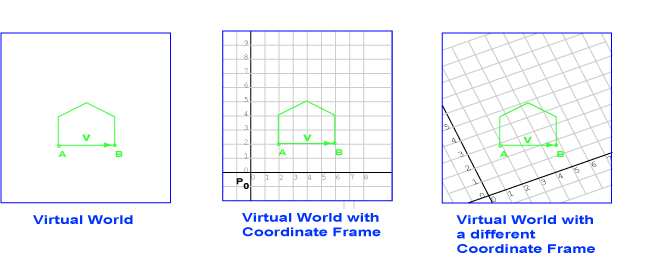Yes.

# Representing Points with Column Matrices

Points can be represented with column matrices. To do this, you first need to decide on a coordinate frame (sometimes called just frame. A coordinate frame consists of a distinguished point P0 (called the origin) and an axis for each dimension (often called X and Y). In 2D space there are two axes; in 3D space there are three axes (often called X, Y, and Z).For now, let us talk about 2D space. The left diagram shows a (rather simple) virtual world in 2D space. The points and vectors exist in the space independent of any coordinate frame. The next diagram shows the same virtual world, this time with a coordinate frame consisting of a particular point P0 and two axes. In this coordinate frame, the point A is represented by the column matrix (2, 2)T.

The right diagram shows the same virtual world, but with a different coordinate frame. In the second coordinate frame, the point A is represented by the column matrix (2, 3)T.

### QUESTION 8:

• How is point B represented using the first coordinate frame?
• How is point B represented using the second coordinate frame?